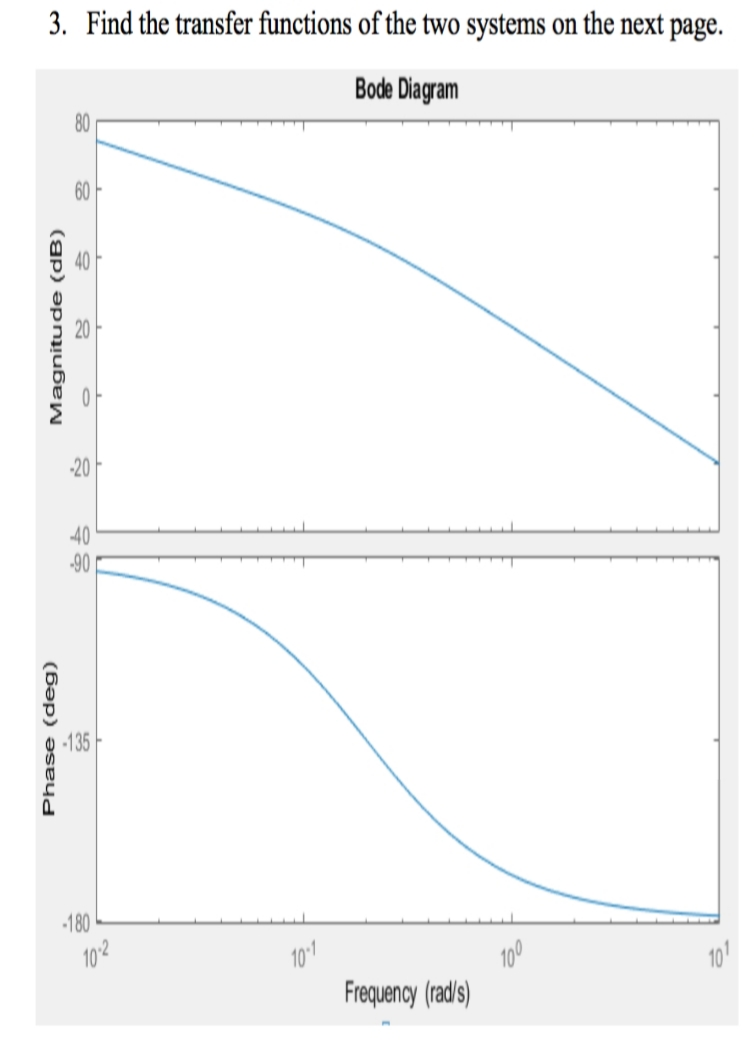# 3. Find the transfer functions of the two systems on the next page.Bode Diagram806040204090135180102101Frequency (rads)10010Phase (deg)Magnitude (dB)

Question
15 viewshelp_outlineImage Transcriptionclose3. Find the transfer functions of the two systems on the next page. Bode Diagram 80 60 40 20 40 90 135 180 102 101 Frequency (rads) 100 10 Phase (deg) Magnitude (dB) fullscreen
check_circle

Step 1

Relative order of system

It is known that the pole of the transfer function at high frequencies contribute -90 degree to the phase and the zero of the transfer functions contribute +90 degree. It is seen from the phase diagram of the bode plot that phase diagram is ending at -180 degree. This clearly shows that transfer function contains 2 more pole than zero.

Step 2

Number of Poles and zeros

To decide the number of poles and zeros of the transfer function, analyse the magnitude plot of the bode plot.

Initially the slope of magnitude is -20 dB/dec and it ends with slope of -40 dB/dec. This shows that transfer function contains two poles and no zeros.

Step 3

Location of Poles and zeros

From the magnitude plot, it is found out that the slope of plot starts by -20 dB/dec, so the transfer function will be type 1 system and one pole will be at 0.

From the phase diagram, plot start at -90 degree. To find out the second slope of the transfer function, extend the asymptotic lines at the be...

### Want to see the full answer?

See Solution

#### Want to see this answer and more?

Solutions are written by subject experts who are available 24/7. Questions are typically answered within 1 hour.*

See Solution
*Response times may vary by subject and question.
Tagged in

### Electrical Engineering